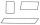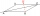# Rhomboid + area of shape - examples

1. ParallelogramCalculate area of the parallelogram ABCD as shown if |AB| = 19 cm, |BC| = 18 cm and angle BAD = 90°
2. RhomboidCalculate the circumference and area of the rhomboid with sides 20 and 10, with their angle 20°.
3. Black buildingKeith built building with a rectangular shape 6.5 m × 3.9 m. Calculate how much percent exceeded the limit 25 m2 for small building. Building not built in accordance with the law is called "black building". Calculate the angle that the walls were clenchin
4. Trapezoid MO-5-Z8ABCD is a trapezoid that lime segment CE divided into a triangle and parallelogram as shown. Point F is the midpoint of CE, DF line passes through the center of the segment BE and the area of the triangle CDE is 3 cm2. Determine the area of the trapezoid A
5. Tetrahedral prismCalculate surface and volume tetrahedral prism, which has a rhomboid-shaped base, and its dimensions are: a = 12 cm, b = 7 cm, ha = 6 cm and prism height h = 10 cm.
6. Dusana) Dusan break two same window, which has triangular shape with a length of 0.8 m and corresponding height 9.5 dm. Find how many dm2 of glass he needs to buy for glazing of these windows. b) Since the money to fix Dusan has not, must go to the paint job
7. RhumblineFind circumference and area of the rhumbline ABCD if the short side AD of which has a length of 5 cm, and the heel of the height from D leading to the AB side divides the AB side into two sections of 3 cm and 4 cm.
8. ParallelogramRhomboid (parallelogram) has a longer side of 50 cm long. The size of its one height is four times the size of its second height. Calculate the length of the shorter side of this rhomboid in the centimeters.

We apologize, but in this category are not a lot of examples.
Do you have an interesting mathematical example that you can't solve it? Enter it, and we can try to solve it.

To this e-mail address, we will reply solution; solved examples are also published here. Please enter e-mail correctly and check whether you don't have a full mailbox.